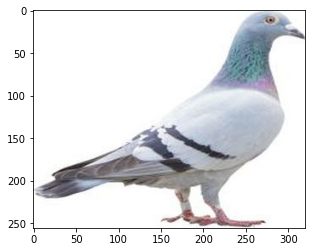• +91-9872993883
• +91-8283824812
• info@ris-ai.com

# Measure similarity between images using Python-OpenCV ¶

In this article , we will predict the images according to their similarities. Image similarity measures play an important role in many applications, such as duplicate product detection, image clustering , visual search etc.

In :
# test image
import matplotlib.pyplot as plt
import matplotlib.image as mpimg
imgplot = plt.imshow(img)
plt.show()In :
img = mpimg.imread('Downloads/peacock.jpg')
imgplot = plt.imshow(img)
plt.show()In :
img = mpimg.imread('Downloads/pegion.jpg')
imgplot = plt.imshow(img)
plt.show()In :
import cv2
# test image
gray_image = cv2.cvtColor(image, cv2.COLOR_BGR2GRAY)
histogram = cv2.calcHist([gray_image], , None, , [0, 256])

# data1 image
gray_image1 = cv2.cvtColor(image, cv2.COLOR_BGR2GRAY)
histogram1 = cv2.calcHist([gray_image1], , None, , [0, 256])

# data2 image
gray_image2 = cv2.cvtColor(image, cv2.COLOR_BGR2GRAY)
histogram2 = cv2.calcHist([gray_image2], , None, , [0, 256])

c1, c2 = 0, 0

# Euclidean Distace between data1 and test
i = 0
while i<len(histogram) and i<len(histogram1):
c1+=(histogram[i]-histogram1[i])**2
i+= 1
c1 = c1**(1 / 2)

i = 0
while i<len(histogram) and i<len(histogram2):
c2+=(histogram[i]-histogram2[i])**2
i+= 1
c2 = c2**(1 / 2)

if(c1<c2):
print("data1.jpg is more similar to test.jpg as compare to data2.jpg")
imgplot1 = plt.imshow(img1)
plt.show()

imgplot = plt.imshow(img)
plt.show()
else:
print("data2.jpg is more similar to test.jpg as compare to data1.jpg")
imgplot1 = plt.imshow(img1)
plt.show()


data2.jpg is more similar to test.jpg as compare to data1.jpg